# constants – physical constants and cosmological calculations¶

The constants module contains attributes storing physical constants and conversion factors. Most of these are at the package level and should be imported as:

from astropysics.constants import c,G,ergperev


The following constants are included (all in cgs units):

• G: Newton’s gravitational constant
• mp: proton mass
• me: electron mass
• e: electron charge
• Ms: solar mass
• Mj: jupiter mass
• Me: earth mass
• Rea: equatorial earth radius (as defined by WGS84)
• Reb: polar earth radius (as defined by WGS84)
• Lsun: solar luminosity
• kb: boltzmann’s constant
• Rb: gas constant
• c: speed of light - exact
• h: planck’s constant
• hbar: reduced planck’s constant
• g0: mean earth gravitational acceleration at sea level

The following unit conversion factors are also provided:

• ergperev
• secperday
• secperyr
• secpergyr
• cmperpc
• pcpercm
• lyperpc
• pcperly
• cmperau
• aupercm

Additional, convinience or derived values include:

• GMskm: Standard gravitational parameter for the sun in km^3 s^-2
• GMsau: Standard gravitational parameter for the sun in AU km^2 s^-2
• GMspc: Standard gravitational parameter for the sun in pc km^2 s^-2

The package also includes classes representing various cosmologies that are used to derive relevant cosmological parameters. The current default is the WMAP7Cosmology, based on the LCDM cosmology with parameters favored by WMAP7 .

The cosmological parameters for the builtin cosmologies are:

• H0: Hubble’s constant (all cosmologies)
• h: H0/100 (all cosmologies)
• h7: H0/70 (all cosmologies)
• omega: Total energy density as a fraction of the critical density (any FRW)
• omegaR: radiataion desnity (any FRW)
• omegaM: total matter density (any FRW)
• omegaL: dark energy/cosmological constant density (any FRW)
• omegaK: curvature density (any FRW)
• sigma8: rmc density fluctuation amplitude at 8 Mpc/h (WMAP)
• omegaB: Baryon density (WMAP)
• omegaC: dark/non-baryonic matter density (WMAP)
• ns: primordial power spectrum index (WMAP)
• t0: Age of universe in Gyr (WMAP)

The currently active cosmology will export it’s parameters so they should be used in other modules as:

from astropysics.constants import H0,omega


## Classes and Inheritance Structure¶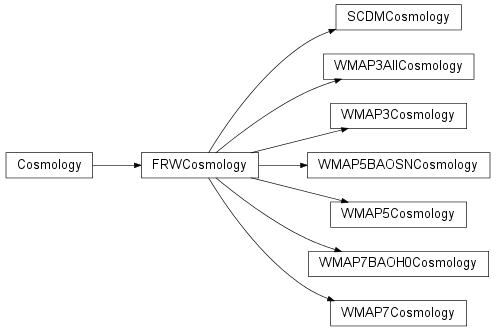## Module API¶

class astropysics.constants.Cosmology

Bases: object

A base class for a cosmology - intended to be subclassed, as this cosmology only has a hubble constant.

Subclassing

• All cosmologies should have a hubble constant (H0) in km/s/Mpc
• Subclasses should also define a class variable _params with a list of strings that specify the names of the cosmological parameters for the subclasses cosmology. These will be exported to the constants module.
• Error bars for parameters in _params can optional be specified as a class variable <paramname>_err. These should be a tuple (lowererr,uppererr)
H0 = 0

Hubble constant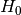for this cosmology in units of km s^-1/Mpc.

getParamWithError(parname)

Returns the requested parameter’s value and its associated errors.

Parameters: parname – The name of the parameter to be retrieved. A tuple (parval,lowererr,uppererr)
h

Reduced Hubble constant: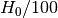.

h7

Reduced Hubble constant: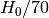.

h70

Reduced Hubble constant:.

params

Names of the cosmological parameters for this Cosmology.

class astropysics.constants.FRWCosmology

A cosmology based on the FRW metric with a global density, a matter density, and a radiation density, and a comological constant as specified at z=0

default values are approximately LambdaCDM

H(z)
H0 = 72
computeOmegaKz(z, units='cgs')

compute curvature density at arbitrary redshift

computeOmegaLz(z)

compute cosmological constant density at arbitrary redshift

computeOmegaMz(z)

compute matter density at arbitrary redshift

computeOmegaRz(z)

compute radiation density at arbitrary redshift

deltavir(z=0)

Virial overdensity asparamaterized in Bryan&Norman 98 for a given redshift.

Good to 1% for omega(z) = 0.1-1, requires either omega = 1 (flat universe) or omega_lambda = 0.

omega
omegaK
omegaL = 0.7
omegaM = 0.3
omegaR = 0
rho(z=0, units='cgs')

mean density in this cosmology

units can be ‘cgs’ or ‘cosmological’ (Mpc,Msun)

rhoC(z=0, units='cgs')

Computes the critical density at a given redshift, e.g.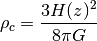Parameters: Returns: z (scalar or array) – redshift at which to compute critical density units (string) – ‘cgs’: g/cm^3 ‘cosmological’: Msun/Mpc^3 Critical density in the requested units if an unrecognized units string is given
class astropysics.constants.SCDMCosmology

“Standard” CDM – flat, with no cosmological constant.

omegaL = 0
omegaM = 1.0
omegaR = 0
class astropysics.constants.WMAP3AllCosmology
H0 = 70.4
H0_err = (1.6, 1.5)
ns = 0.947
ns_err = 0.015
omegaB = 0.04266666666666667
omegaB_err = 0.0013
omegaC = 0.19766666666666668
omegaC_err = (0.0079, 0.0077)
omegaL = 0.7596666666666667
omegaL_err = 0.018
omegaM
omegaM_err
sigma8 = 0.776
sigma8_err = (0.032, 0.031)
class astropysics.constants.WMAP3Cosmology
H0 = 73.2
H0_err = (3.2, 3.1)
ns = 0.958
ns_err = 0.016
omegaB = 0.044
omegaB_err = 0.0014
omegaC = 0.224
omegaC_err = 0.014
omegaL = 0.732
omegaL_err = 0.034
omegaM
omegaM_err
sigma8 = 0.761
sigma8_err = (0.048, 0.049)
class astropysics.constants.WMAP5BAOSNCosmology
H0 = 70.1
H0_err = 1.3
ns = 0.96
ns_err = (0.013, 0.014)
omegaB = 0.046
omegaB_err = 0.0015
omegaC = 0.233
omegaC_err = 0.013
omegaL = 0.721
omegaL_err = 0.015
omegaM
omegaM_err
sigma8 = 0.817
sigma8_err = 0.026
t0 = 13.73
t0_err = 0.12
class astropysics.constants.WMAP5Cosmology
H0 = 71.9
H0_err = (2.7, 2.6)
ns = 0.963
ns_err = (0.015, 0.014)
omegaB = 0.044
omegaB_err = 0.003
omegaC = 0.214
omegaC_err = 0.027
omegaL = 0.742
omegaL_err = 0.03
omegaM
omegaM_err
sigma8 = 0.796
sigma8_err = 0.036
t0 = 13.69
t0_err = 0.13
class astropysics.constants.WMAP7BAOH0Cosmology
H0 = 70.4
H0_err = (1.4, 1.3)
ns = 0.963
ns_err = 0.012
omegaB = 0.045
omegaB_err = 0.0016
omegaC = 0.227
omegaC_err = 0.014
omegaL = 0.728
omegaL_err = (0.016, 0.015)
omegaM
omegaM_err
sigma8 = 0.809
sigma8_err = 0.024
t0 = 13.78
t0_err = 0.11
class astropysics.constants.WMAP7Cosmology
H0 = 71.0
H0_err = 2.5
ns = 0.963
ns_err = 0.014
omegaB = 0.044
omegaB_err = 0.0028
omegaC = 0.222
omegaC_err = 0.026
omegaL = 0.734
omegaL_err = 0.029
omegaM
omegaM_err
sigma8 = 0.801
sigma8_err = 0.03
t0 = 13.71
t0_err = 0.13
astropysics.constants.choose_cosmology(nameorobj, autoupdate=True, args=None, kwargs=None)

Change the currently active cosmology and export its cosmological parameters into the package namespace.

Parameters: nameorobj (string or Cosmology object) – the new cosmology to use autoupdate (bool) – If True, the cosmology object will automatically propogate changes to its parameters up to the module variables. Otherwise, update_cosmology() must be called explicitly to have this behavior occur. args (dictionary or None) – If nameorobj is a string, these are passed in as positional arguments to the object initializer (if not None). Otherwise it is ignored. args – If nameorobj is a string, these are passed in as keyword arguments to the object initializer (if not None). Otherwise it is ignored. the Cosmology object after being assigned as current.
astropysics.constants.flambda_to_fnu_l(flambda, lamb)
astropysics.constants.flambda_to_fnu_n(flambda, nu)
astropysics.constants.fnu_to_flambda_l(fnu, lamb)
astropysics.constants.fnu_to_flambda_n(fnu, nu)
astropysics.constants.get_cosmology(name=None)

If name is None, will retreive the currently in use Cosmology instance. Otherwise, returns the subclass of Cosmology with the provided name.

astropysics.constants.get_registry_names()

Returns the names of all cosmology types in the registry

astropysics.constants.register_cosmology(cosmocls, name=None)

Add the provided subclass of Cosmology to the cosmology registry

if name is None, the name will be inferred from the class name, otherwise

astropysics.constants.update_cosmology()

updates the package-level variables for changes in the current Cosmology object